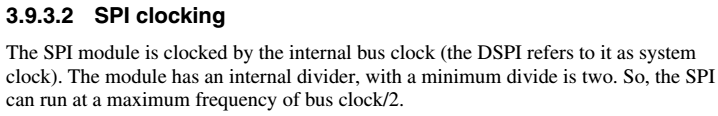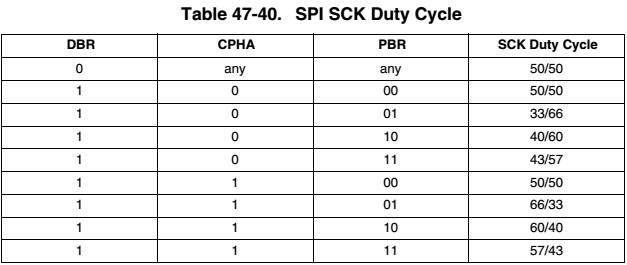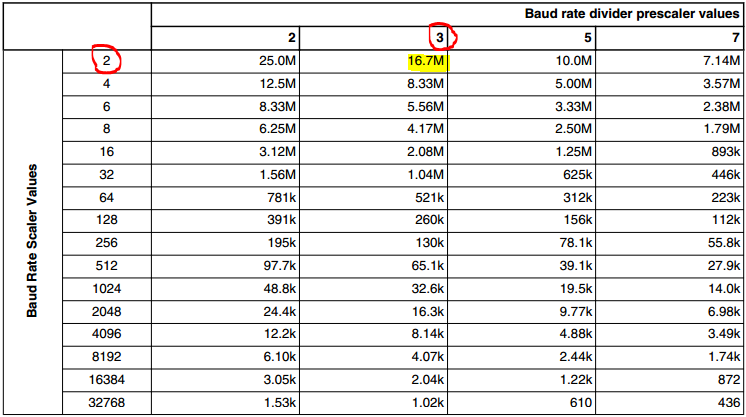## Duty cycle in Kinetis SPI

cancel
Showing results for
Search instead for
Did you mean:
SOLVED

## Duty cycle in Kinetis SPI

Jump to solution
1,169 ViewsNXP Employee

Hi,

I am using K22 uC at max 120Mhz clock and would like to run SPI at @speed of 20MHz with 50/50 duty cycle. The max speed for SPI at 120MHz clock is 30MHz while through double baud rate(multiplied by 1 or 2), baud rate prescalers (2,3,5 and 7) and scalers (2,4,6,8,16,32,64,...), it can be dropped down to several other options. But for the cases when prescaler is not 2 and double baud rate is enabled, the duty cycle varies and is not 50/50 anymore.

Is there a way that I can run SPI for bus speed of 20MHz with 50/50 duty cycle?

Regards,

Saad

Labels (3)

• ### Kinetis K Series MCUs

Tags (3)
1 Solution
319 ViewsSenior Contributor II

Hello,

Do you have errors because the duty cycle is not 50%?

The Fp (frequency protocol) is the same as your bus clock, I suppose that you have a bus clock running at 60 MHz if your core clock is 120 MHz so you can not obtain a 20 MHz SPI clock if the DBR bit is cleared.As we reviewed in this message​, to obtain a 20 MHz sck signal since a bus clock of 60 MHz, the relation PBR*BR must be 6 and DBR must be set. It can be seen in the table below that if the DBR bit is set, only the SCK duty cycle will be 50% if the PBR (Baud Rate Prescaler) is 00 (Baud Rate Prescaler value is 2 if PBR = 0). So if you configure PBR = 00 and DBR = 1 and you want a SCK duty cycle of 50%, the Baud Rate scaler must be 3 which is not possible.Regards,

Earl.

5 Replies
319 ViewsSenior Contributor II

Hello Saad,

The table 47-73 in the page 1297 in the K22 Reference Manual​ shows the baud rate generated based on the combination of the PBR (prescaler) and the BR (scaler) registers. This table assumes that the bit DBR is cleared and a 100 MHz protocol frequency. If your system is running 1.2 times faster than 100 MHz then you can use PBR = 3 and BR = 2 to get 20 MHz (20 MHz is 1.2 times faster than 16.7 MHz). Does this work aligned with your requirements?Best regards,

Earl Orlando.

319 ViewsNXP Employee

Hi Earl Orlando,

The formula used on pg. 1259 of the Reference Manual uses this formula for calculation of baud rate: SCK baud rate = (Fp /PBR) x [(1+DBR)/BR]. I have used Fp as max bus speed (half of clock speed) which is 60MHz for 120MHz clock. However it seems from the chart [you have posted] that Fp is equal to 120MHz for 120MHz clock. Would you please tell what exactly Fp is, for 120MHz clock?

P.S: Seems like, with 120MHz (max) clock and PBR=2, BR=2 and with DBR set we can achieve max SPI speed of 60 MHz. (Is it correct?)

Regards,

Saad

319 ViewsSenior Contributor II

Sorry, I was wrong. According to the section 3.9.3.2, the SPI module is fed by the bus clock (in this case 60 MHz maximum), this is the Fp (frequency protocol). According to the baud rate formula SCK baud rate = (Fp /PBR) x [(1+DBR)/BR] = 20 MHz = 60 MHz * [(1 + DBR) / (PBR * BR)], if you want a 20 MHz frequency you need that the relation [(1 + DBR) / (PBR * BR)] = 1 / 3, if you clear the DBR bit the relation PBR * BR = 3 which seems to be impossible. But if you set the DBR bit, the relation (1 + DBR) / (PBR * BR) = 2 / (PBR * BR) = 1 / 3 and PBR * BR = 6. If you make this configuration, is not the SCK's duty cycle 50%?

Earl.

319 ViewsNXP Employee

Hi, for all those configurations when DBR is set and PBR is not equal to 2, the duty cycle is not 50/50 and in this case as well the duty cycle will not be 50%. The chart you shared, suggests that Fp=100MHz with DBR cleared. Is this frequency not possible with current device (K22 120MHz)?

Regards,

Saad

320 ViewsSenior Contributor II

Hello,

Do you have errors because the duty cycle is not 50%?

The Fp (frequency protocol) is the same as your bus clock, I suppose that you have a bus clock running at 60 MHz if your core clock is 120 MHz so you can not obtain a 20 MHz SPI clock if the DBR bit is cleared.As we reviewed in this message​, to obtain a 20 MHz sck signal since a bus clock of 60 MHz, the relation PBR*BR must be 6 and DBR must be set. It can be seen in the table below that if the DBR bit is set, only the SCK duty cycle will be 50% if the PBR (Baud Rate Prescaler) is 00 (Baud Rate Prescaler value is 2 if PBR = 0). So if you configure PBR = 00 and DBR = 1 and you want a SCK duty cycle of 50%, the Baud Rate scaler must be 3 which is not possible.Regards,

Earl.